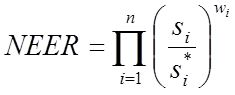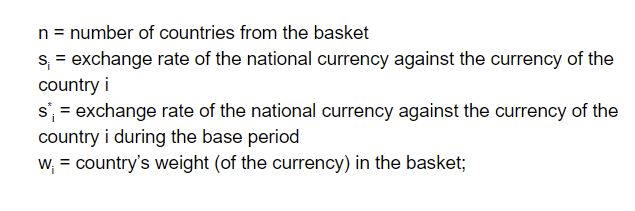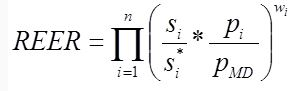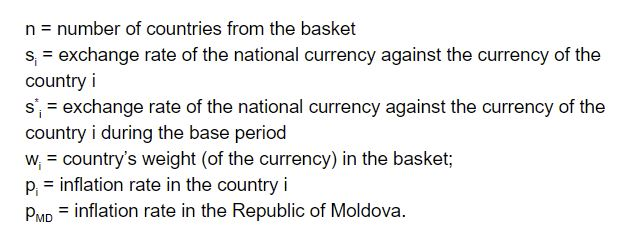# NEER and REER - Difference between Reer & Neer (UPSC Notes)

Nominal Effective Exchange Rate (NEER) and Real Effective Exchange Rate (REER) are indicators of external competitiveness. Neer is a weighted index that reflects the trade of India with other countries. The weight is greater for countries with which India trades more. Reer is again a weighted index which also includes domestic inflation in various economies. Read the facts about Reer and Neer in this article for the IAS Exam, as the topic comes under Prelims (Indian Economy) and Mains GS-III.

 To complement your UPSC GS III Preparation, check the following links:

## What is NEER?

The nominal effective exchange rate (NEER) is an unadjusted weighted average rate at which one country’s currency exchanges for a basket of multiple foreign currencies. The nominal exchange rate is the amount of domestic currency needed to purchase foreign currency.

In economics, the NEER is an indicator of a country’s international competitiveness in terms of the foreign exchange (forex) market. Forex traders sometimes refer to the NEER as the trade-weighted currency index.

The NEER may be adjusted to compensate for the inflation rate of the home country relative to the inflation rate of its trading partners. The resulting figure is the real effective exchange rate (REER). Unlike the relationships in a nominal exchange rate, NEER is not determined for each currency separately. Instead, one individual number, typically an index, expresses how a domestic currency’s value compares against multiple foreign currencies at once.

How to calculate NEER?

The Nominal Effective Exchange Rate can be calculated from the following formula:## What is Real Effective Exchange Rate (REER)?

The real effective exchange rate (REER) is the weighted average of a country’s currency in relation to an index or basket of other major currencies. The weights are determined by comparing the relative trade balance of a country’s currency against each country within the index.

This exchange rate is used to determine an individual country’s currency value relative to the other major currencies in the index.

The formula used for calculating REER is mentioned below:How to calculate REER?

• A country’s REER can be derived by taking the average of the bilateral exchange rates between itself and its trading partners and then weighing it using the trade allocation of each partner.
• The average of the exchange rates is calculated after assigning the weightings for each rate. For example, if a currency had a 60% weighting, the exchange rate would be raised to the power by 0.60 and do the same for each exchange rate and its respective weighting.
• Multiply each exchange rate in step 2 by each other and multiply the final result by 100 to create the scale or index.
• Some calculations use bilateral exchange rates while other models use real exchange rates, which adjusts the exchange rate for inflation. Regardless of the way in which REER is calculated, it is an average and considered in equilibrium when it is overvalued in relation to one trading partner and undervalued in relation to a second partner.